# shapviz

## Introduction

SHAP (SHapley Additive exPlanations, see Lundberg and Lee (2017)) is an ingenious way to study black box models. SHAP values decompose - as fair as possible - predictions into additive feature contributions. Crunching SHAP values requires clever algorithms by clever people. Analyzing them, however, is super easy with the right visualizations. The “shapviz” package offers the latter:

• sv_dependence(): Dependence plots to study feature effects (optionally colored by heuristically strongest interacting feature).
• sv_importance(): Importance plots (bar plots and/or beeswarm plots) to study variable importance.
• sv_waterfall(): Waterfall plots to study single predictions.
• sv_force(): Force plots as an alternative to waterfall plots.

These plots require a “shapviz” object, which is built from two things only:

1. S: Matrix of SHAP values
2. X: Dataset with corresponding feature values

Furthermore, a baseline can be passed to represent an average prediction on the scale of the SHAP values.

A key feature of “shapviz” is that X is used for visualization only. Thus it is perfectly fine to use factor variables, even if the underlying model would not accept these. Additionally, in order to improve visualization, it can sometimes make sense to clip gross outliers, take logarithms for certain columns, or replace missing values by some explicit value.

To further simplify the use of “shapviz”, we added direct connectors to the R packages

CatBoost is not included, but see Section “Any other package” how to use its SHAP calculation backend with “shapviz”.

## Installation

# From CRAN
install.packages("shapviz")

# Or the newest version from GitHub:
# install.packages("devtools")
devtools::install_github("mayer79/shapviz")

## Example: Diamond prices

### Fit model

We start by fitting an XGBoost model to predict diamond prices based on the four “C” features.

library(shapviz)
library(ggplot2)
library(xgboost)

set.seed(3653)

ord <- c("clarity", "cut", "color")
diamonds[, ord] <- lapply(diamonds[, ord], factor, ordered = FALSE)

X <- diamonds[c("carat", "cut", "color", "clarity")]
dtrain <- xgb.DMatrix(data.matrix(X), label = diamonds$price) fit <- xgb.train( params = list(learning_rate = 0.1, objective = "reg:squarederror"), data = dtrain, nrounds = 65L ) ### Create “shapviz” object One line of code creates a “shapviz” object. It contains SHAP values and feature values for the set of observations we are interested in. Note again that X is solely used as explanation dataset, not for calculating SHAP values. In this example we construct the “shapviz” object directly from the fitted XGBoost model. Thus we also need to pass a corresponding prediction dataset X_pred used for calculating SHAP values by XGBoost. X_small <- X[sample(nrow(X), 2000L), ] # X is the "explanation" dataset using the original factors shp <- shapviz(fit, X_pred = data.matrix(X_small), X = X_small) Note: If X_pred would contain one-hot-encoded dummy variables, their SHAP values could be collapsed by the collapse argument of shapviz(). ### Decompose single prediction The main idea behind SHAP values is to decompose, in a fair way, a prediction into additive contributions of each feature. Typical visualizations include waterfall plots and force plots: sv_waterfall(shp, row_id = 1L) + theme(axis.text = element_text(size = 11))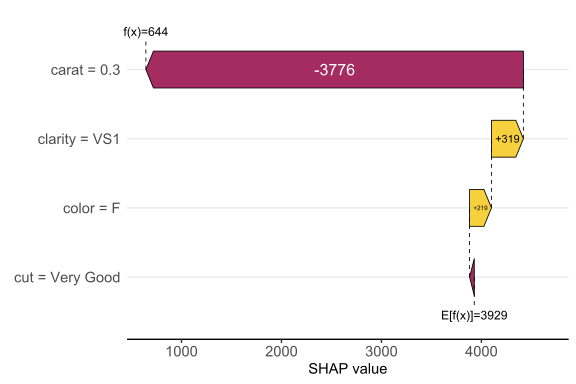Works pretty sweet, and factor input is respected! Alternatively, we can study a force plot: sv_force(shp, row_id = 1L)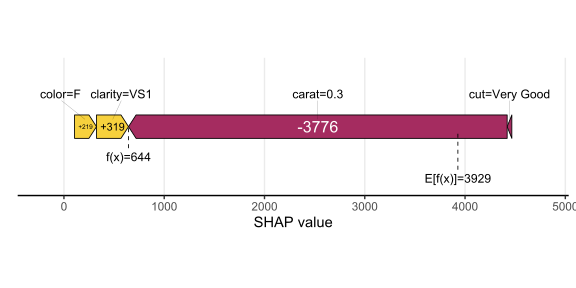### SHAP importance Studying SHAP decompositions of many observations allows to gain an impression on variable importance. As simple descriptive measure, the mean absolute SHAP value of each feature is considered. These values can be plotted as a simple bar plot, or, to add information on the sign of the feature effects, as a beeswarm plot sorted by the mean absolute SHAP values. Such beeswarm plots are often called “summary plots”. # A bar plot of mean absolute SHAP values sv_importance(shp)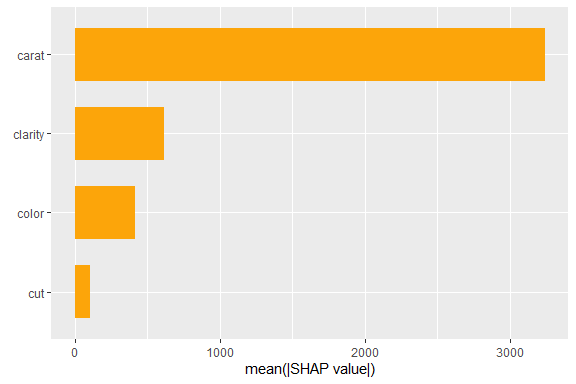# A beeswarm plot sv_importance(shp, kind = "beeswarm")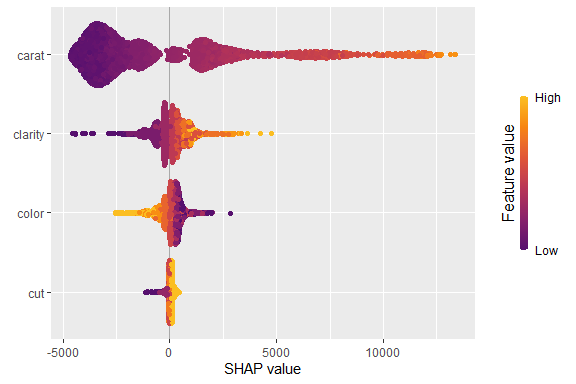# Or both! sv_importance(shp, kind = "both", show_numbers = TRUE, width = 0.2)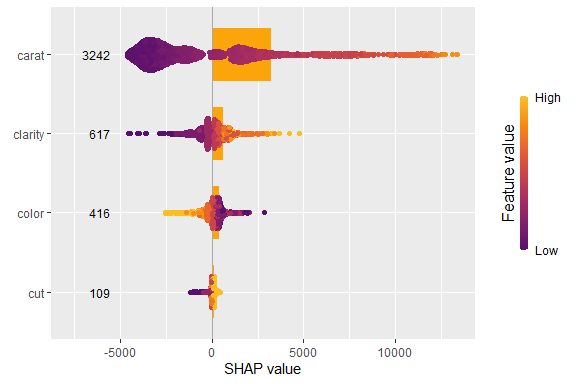### SHAP dependence plots A SHAP beeswarm importance plot gives first hints on whether high feature values tend to high or low predictions. This impression can be substantiated by studying simple scatterplots of SHAP values of a feature against its feature values. A second feature can be added as color information to see whether the feature effect depends on the feature on the color scale or not. The stronger the vertical scatter for similar values on the x axis, the stronger the interactions. sv_dependence(shp, v = "color", color_var = "auto")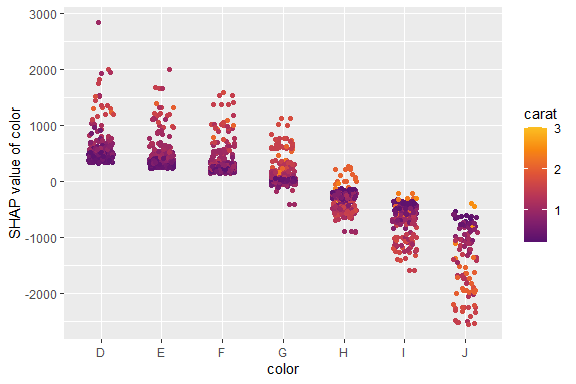sv_dependence(shp, v = "carat", color_var = "auto", alpha = 0.2, size = 1) + guides(colour = guide_legend(override.aes = list(alpha = 1, size = 2)))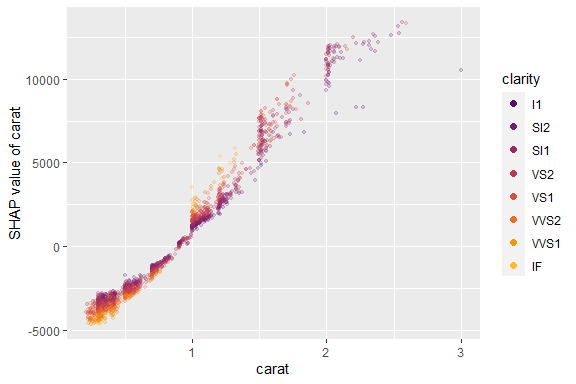## Interface to other packages The above example uses XGBoost to calculate SHAP values. In the following sections, we show (without running the code), how other packages work together with “shapviz”. ### LightGBM library(lightgbm) dtrain <- lgb.Dataset(data.matrix(X), label = diamonds$price)

fit <- lgb.train(
params = list(learning_rate = 0.1, objective = "regression"),
data = dtrain,
nrounds = 65L
)

shp <- shapviz(fit, X_pred = data.matrix(X_small), X = X_small)
sv_importance(shp)

### fastshap

library(fastshap)

fit <- lm(price ~ carat + clarity + cut + color, data = diamonds)
explainer <- explain(fit, newdata = X_small, exact = TRUE)
shp <- shapviz(explainer, X = X_small)
sv_dependence(shp, "carat")

### shapr

library(shapr)

fit <- lm(price ~ carat + clarity + cut + color, data = diamonds)
X_smaller <- X[sample(nrow(X), 200L), ]

explainer <- shapr(X_smaller, fit,)

# Takes about 15 seconds
explanation <- explain(
X_smaller,
approach = "ctree",
explainer = explainer,
prediction_zero = mean(diamonds$price) ) shp <- shapviz(explanation) sv_dependence(shp, "carat", "auto") ### H2O If you work with a tree-based H2O model: library(h2o) h2o.init() dia_h2o <- as.h2o(diamonds) fit <- h2o.gbm(c("carat", "clarity", "color", "cut"), "price", training_frame = dia_h2o) x <- shapviz(fit, X_pred = X_small) sv_force(x, row_id = 1) sv_dependence(x, "carat", "auto") ### treeshap library(treeshap) library(ranger) fit <- ranger(y = diamonds$price, x = data.matrix(X), max.depth = 6, num.trees = 100)
unified_model <- ranger.unify(fit, data.matrix(X))
shaps <- treeshap(unified_model, data.matrix(X_small))
shp <- shapviz(shaps, X = X_small)
sv_importance(shp)
sv_dependence(shp, "clarity", color_var = "auto", alpha = 0.2, size = 1)

### kernelshap

library(kernelshap)

X_smaller <- X[sample(nrow(X), 200L), ]
fit <- lm(price ~ carat + clarity + cut + color, data = diamonds)

# Takes about 30 seconds
system.time(
ks <- kernelshap(X_smaller, function(X) predict(fit, X), X_smaller)
)

shp <- shapviz(ks)
sv_importance(shp)
sv_dependence(shp, "carat")

### Any other package

The most general interface is to provide a matrix of SHAP values and corresponding feature values (and optionally, a baseline value):

S <- matrix(c(1, -1, -1, 1), ncol = 2, dimnames = list(NULL, c("x", "y")))
X <- data.frame(x = c("a", "b"), y = c(100, 10))
shp <- shapviz(S, X, baseline = 4)

An example is CatBoost: it is not on CRAN and requires catboost.*() functions to calculate SHAP values, so we cannot directly add it to “shapviz” for now. Just use a wrapper like this:

library(catboost)

shapviz.catboost.Model <- function(object, X_pred, X = X_pred, collapse = NULL, ...) {
if (!requireNamespace("catboost", quietly = TRUE)) {
stop("Package 'catboost' not installed")
}
stopifnot(
"X must be a matrix or data.frame. It can't be an object of class catboost.Pool" =
is.matrix(X) || is.data.frame(X),
"X_pred must be a matrix, a data.frame, or a catboost.Pool" =
is.matrix(X_pred) || is.data.frame(X_pred) || inherits(X_pred, "catboost.Pool"),
"X_pred must have column names" = !is.null(colnames(X_pred))
)

if (!inherits(X_pred, "catboost.Pool")) {
}

S <- catboost.get_feature_importance(object, X_pred, type = "ShapValues", ...)

# Call matrix method
pp <- ncol(X_pred) + 1L
baseline <- S[1L, pp]
S <- S[, -pp, drop = FALSE]
colnames(S) <- colnames(X_pred)
shapviz(S, X = X, baseline = baseline, collapse = collapse)
}

# Example

X_pool <- catboost.load_pool(diamonds[colnames(X)], label = diamonds\$price)

fit <- catboost.train(
X_pool,
params = list(
loss_function = "RMSE",
iterations = 165,
logging_level = "Silent",
allow_writing_files = FALSE
)
)

shp <- shapviz(fit, X_small)
sv_importance(shp)
sv_dependence(shp, "clarity", color_var = "auto", alpha = 0.2, size = 1)

## Classification models

The plot functions work with one-dimensional model predictions only. However, the wrappers for XGBoost and LightGBM allow to select the category of interest and work with its predicted (logit) probabilities, simply by providing which_class in the constructor.